# Chapter 6The ECG Recording: Leads, Devices, and Techniques

The ECG waves are recorded in different forms according to the location of the explorer electrode (lead). The morphology of the ECG is determined by the location at which the lead is recorded. Because the heart is a three‐dimensional organ, it is necessary to know the projection of the electrical activity at least in two planes—the frontal plane and the horizontal plane—to determine the exact direction of the different vectors that express the electrical forces of the heart. To record these forces, cables that connect the patient with the galvanometer of the ECG device are necessary, which pass through an amplifier. These cables are connected to the skin through special plaques (see Figure 6.19). ECG devices record the electrical activity of the heart through both frontal and horizontal plane leads. There are six frontal plane leads and six horizontal plane leads (V1–V6).

Just as it is necessary to photograph a monument or person from various angles in order to obtain comprehensive information about its size and shape (Figure 6.1), the electrical forces generated by the heart need to be recorded from different places (leads) to obtain a good electrocardiographic image of the heart. Each lead has a lead line which goes from the place where the lead is located to the opposite zone, passing through the center of the heart. Each of the lead lines is divided into two segments: the positive half, which is proximal to the positive pole and indicated by a continuous line, and the negative half, which is proximal to the negative pole and indicated by a dotted line.

#### Standard limb leads: I, II, III

The three standard limb leads record the potential differences between two parts of the body. They constitute a closed circuit (Figure 6.2). Einthoven designated these leads as I, II, and III. To record these leads, electrodes are placed on the right arm, left arm, and left foot (Figure 6.2). These bipolar leads reveal the direction and magnitude of the vectorial forces in the frontal plane (up–down, right–left).The potential differences recorded by these leads are explained in the legend of Figure 6.3.

The needle of the electrocardiograph inscribes the electrical forces as positive upward deflections () or negative, downward deflections (), or diphasic complexes, such as complexes consisting of both a positive–negative or negative–positive deflection (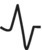,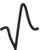).

A positive deflection (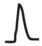) in a certain lead represents the electrical forces pointed toward the positive electrode of this lead; a negative deflection (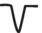) represents electrical forces pointed toward the negative electrode of the same lead (Figure 6.3). An isodiphasic (,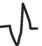) deflection indicates that the vectorial forces are perpendicular to the lead line (i.e. they lie on a line separating the positive and negative hemifields of the lead). The correct morphology (+− or −+ may be determined according to whether the rotation of the loop is clockwise or counterclockwise (see Figures 1.5, 1.6, 6.16, and 6.17). A deflection that has the same positive and negative height but different areas () is not considered isodiphasic; a deflection with some positive and negative areas but different heights () is considered isodiphasic.

In accordance with Kirchoff’s law, Einthoven considered these three bipolar leads to be a closed circuit. Lead II = I + III is known as Einthoven’s law. This law should always be satisfied in electrocardiography and is used as means of verifying proper lead placement and labelling.

Using the aforementioned law, Einthoven described an imaginary equilateral triangle superimposed on the human body as a useful visual tool, using the basic idea that electrode placement further down on the arms or legs (Figure 6.4A) is equivalent to placement on the root of the limbs (right shoulder, left shoulder, and groin) (Figure 6.4B). Although the expression of Einthoven’s law as an equilateral triangle is not completely correct—it is evident that the human trunk cannot be considered a homogeneously conductive sphere with the heart at its center (Figure 6.5)—it is the easiest way to remember the basic concepts of ECG (hemifield, distance in angles between leads, calculation of the electrical axis, etc.). According to Einthoven’s triangle, the six leads of the frontal plane form a hexagonal reference system that divides the electrical field into 12 parts separated by 30°. The scalene triangle described by Burger is probably more exact (Figure 6.6). If we follow this, the 12 parts are separated by different angles. However, on some occasions, we may explain some aspects of the ECG–VCG correlation using Burger’s triangle. Figure 11.35, for example, shows that in cases of superoanterior hemiblock the last part of the frontal loop is located beyond −90° but in spite of that we do not record the “S” wave in lead I, because the positive hemifield, according Burger’s triangle (dotted line around −10° in Figure 11.35), really finishes beyond −90°.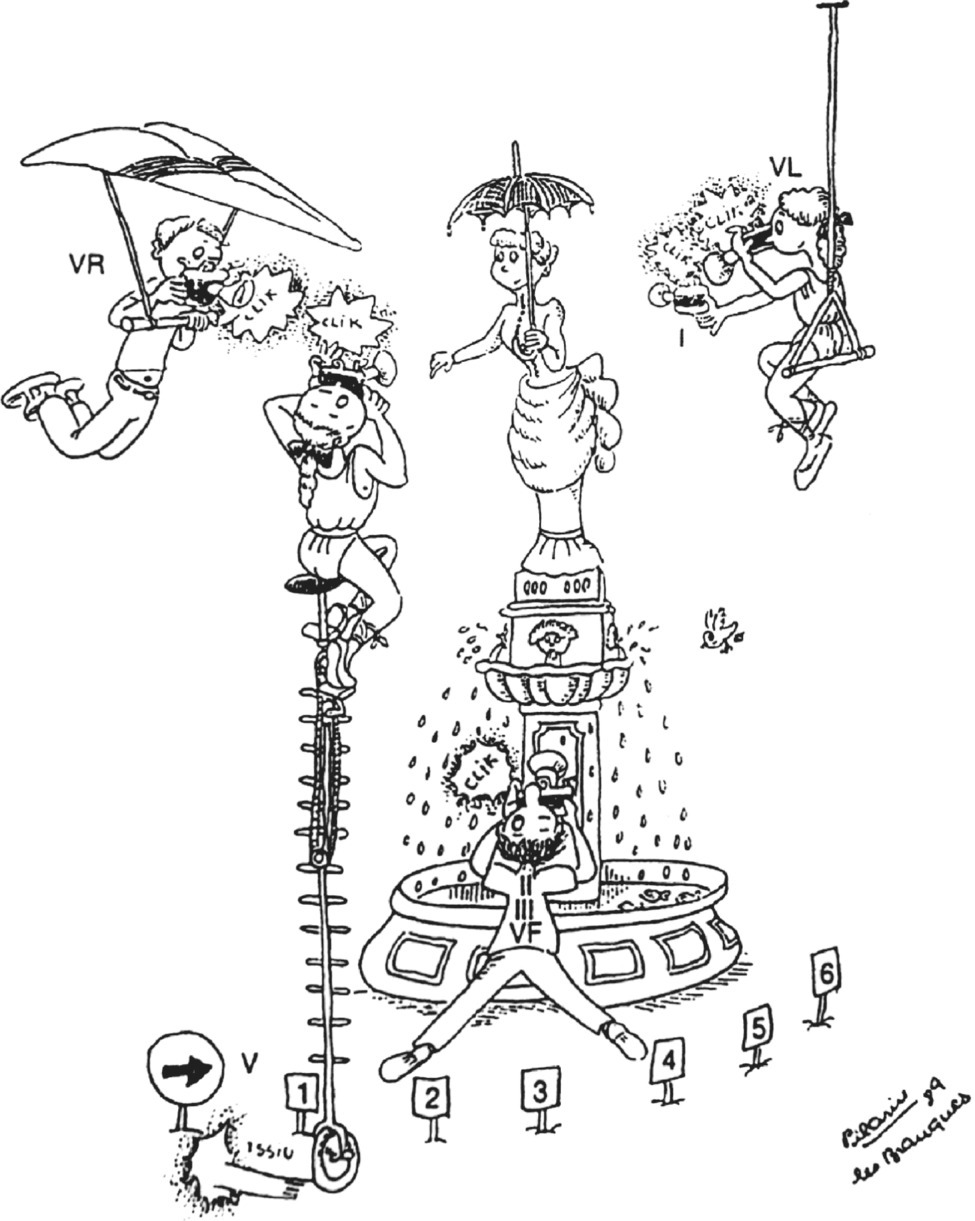Figure 6.1 For a better understanding of a landscape, building, or statue, it is necessary to contemplate or take photographs from different angles, as shown in this case with the “Dama de la Sombrilla” (Umbrella Lady), a landmark in Barcelona. Similarly, if we want to learn about the electrical activity of the heart, it is necessary to record the activation route from different angles (leads) ( drawing by Pilarín Bayés de Luna).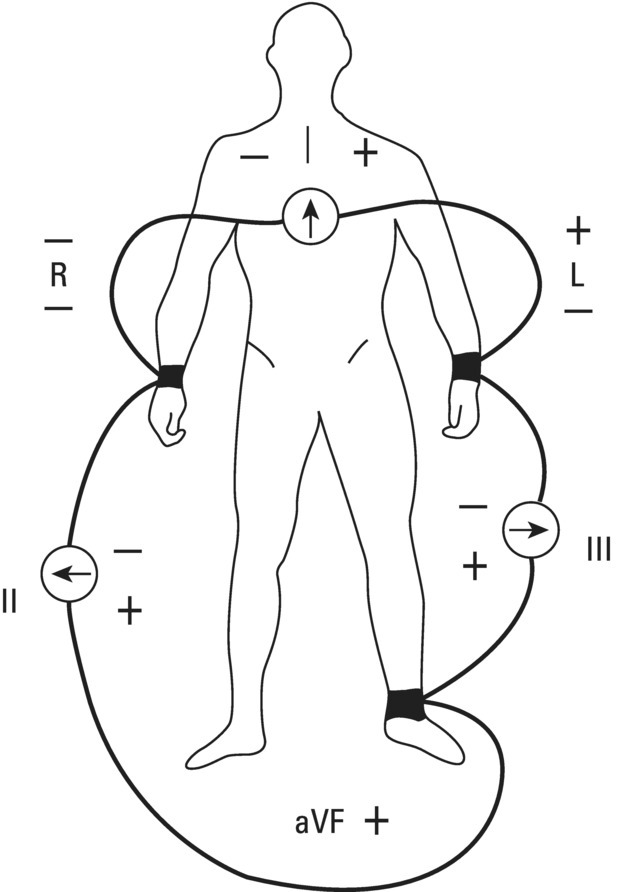Figure 6.2 Human silhouette with the wires placed for the three classic frontal plane leads I, II, and III.

Different vectors generated in the heart (i.e. Vectors 1–6 in Figure 6.4C) result in different projections (positive, negative, and diphasic) on the three sides of the triangle (on the three standard leads of the frontal plane).

Moving the three segments of Einthoven’s triangle (I, II, III) until they intersect at the triangle center, Bailey obtained a reference system (Bailey’s triaxial system) (Figure 6.7) that does not alter the mathematical relationship between leads, I, II, and III, but facilitates the understanding of the positive and negative hemifield concepts. The frontal plane where the reference triaxial figure is situated is divided by the three leads into six spaces (Bailey’s sextants). The positive pole of lead I corresponds to 0°, that of lead II to +60°, and that of lead III to +120°. The negative poles of these leads correspond to ±180°, −120°, and −60°, respectively. Each sextant represents a 60° arc.

#### Limb leads: right and left arm and left foot (aVR, aVL, aVF)

Despite the usefulness of the three bipolar standard limb leads, they reflect only the differences of potential and not the real direct potential in one specific point of the body. In order to separate the two components of standard limb leads, Wilson et al. (1943) joined the three vertices of the Einthoven triangle (right and left arm and left foot) with resistances of 5000 Ω to one point we refer to as the central terminal, obtaining at this point a potential zero (Figure 6.8A). Later, he connected the explorer electrode to the right (R) and left arm (L) and left foot (F) and obtained the absolute net potentials of these three members (right arm, left arm, and left foot): the aVR, aVL, and aVF leads. Although Wilson called these “unipolar” limb leads, really they are also bipolar because they measure differences of potential between one point and the central terminal (AHA/ACC/HRS Guidelines, Kligfield et al. 2007).

To obtain better voltage complexes, Goldberger modified the Wilson system to an augmented system leads (aVR, aVL, aVF) (Figure 6.8B). These three “unipolar” leads are directed from the vertices of the three angles of Einthoven’s triangle (aVR, aVL, aVF) toward the midpoint of the opposite site, intersecting the center of the triangle (Figure 6.9). Each lead has a positive pole extending from the angle of the triangle that coincides with the respective edge to the center of the triangle, and a negative pole, extending practically from the center of the triangle to the midpoint of the opposite side of the triangle. The negative pole of each lead is situated 180° opposite the positive pole. The direction of the lead line of aVR extends from −150° (positive pole) to +30° (negative pole); the lead line of aVL from −30° (positive pole) to +150° (negative pole); and the lead line of VF from +90° (positive pole) to −90° (negative pole).Figure 6.6 Burger’s scalene triangle, which represents the electrical field of the human body more accurately than Einthoven’s triangle.

Using the locations of the three limb leads (triaxial reference system) and combining the axes of the aVF, aVL, and aVF leads with Bailey’s triaxial reference system so that the intersection of the six axes is in the center of the heart, a single figure is obtained which shows the directions, the positive and negative poles, and positive (continuous line) and negative (dotted line) parts of the six frontal plane leads (Bailey’s hexaxial system). In this system (Figure 6.10), leads are separated by 30° angles.

There is an increased interest in revising the standard presentation of the frontal plane ECG leads by changing the lead aVR location from −150° to +30° (Case and Moss 2010). This reorients the frontal plane leads from III to aVL into a progressive logically panoramic sequence that follows the same pattern as the horizontal precordial leads from V1 to V6 (Figure 6.11).Get Clinical Tree app for offline access